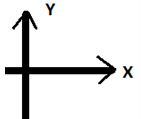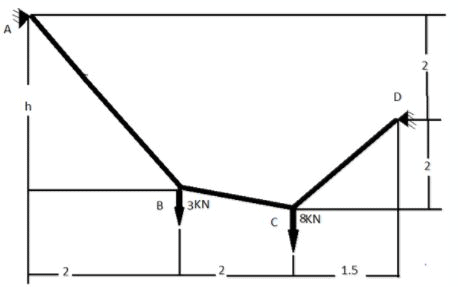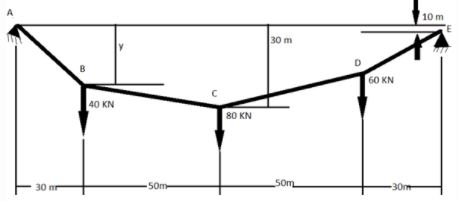Courses

# Test: Cable Subjected to Concentrated Loads & Cables

## 12 Questions MCQ Test Structural Analysis | Test: Cable Subjected to Concentrated Loads & Cables

Description
This mock test of Test: Cable Subjected to Concentrated Loads & Cables for Civil Engineering (CE) helps you for every Civil Engineering (CE) entrance exam. This contains 12 Multiple Choice Questions for Civil Engineering (CE) Test: Cable Subjected to Concentrated Loads & Cables (mcq) to study with solutions a complete question bank. The solved questions answers in this Test: Cable Subjected to Concentrated Loads & Cables quiz give you a good mix of easy questions and tough questions. Civil Engineering (CE) students definitely take this Test: Cable Subjected to Concentrated Loads & Cables exercise for a better result in the exam. You can find other Test: Cable Subjected to Concentrated Loads & Cables extra questions, long questions & short questions for Civil Engineering (CE) on EduRev as well by searching above.
QUESTION: 1

### Following sign convention for force direction is followed:-For moments, clockwise is considered –ve. All the options are given in KN and KN/ft. wherever applicable. All distances mentioned below are in ft. Following is a discreetly loaded cable.Q. Find the tension in cable CD.

Solution:

Explanation: Conserve moment about point A and assume required tension a variable. Bifurcate it into x and y directions to make calculation easier.

QUESTION: 2

### Following sign convention for force direction is followed:-For moments, clockwise is considered –ve. All the options are given in KN and KN/ft. wherever applicable. All distances mentioned below are in ft. Following is a discreetly loaded cable.Q. What will be the tension in cable BC?

Solution:

Explanation: Balance force at point C in x and y direction which will give two equations. Assume the angle between BC and x axis as a variable.

QUESTION: 3

### ​Following sign convention for force direction is followed:-For moments, clockwise is considered –ve. All the options are given in KN and KN/ft. wherever applicable. All distances mentioned below are in ft. Following is a discreetly loaded cable.Q. What will be the tension in cable AB?

Solution:

Explanation: Balance force at point B in x and y direction which will give two equations. Assume the angle between AB and x axis as a variable.

QUESTION: 4

​Following sign convention for force direction is followed:-For moments, clockwise is considered –ve.
All the options are given in KN and KN/ft. wherever applicable.
All distances mentioned below are in ft.
Following is a discreetly loaded cable.Q. What will be the value of h?

Solution:

Explanation: Since all the angles are known, we can find h using basic trigonometry.

QUESTION: 5

All the options are given in KN and KN/m. wherever applicable.
All distances mentioned below are in m.
Following is a discreetly loaded cable.
A and E are supported by pin supports.Q. Compute tension in cable AB.

Solution:

Explanation: Balance moment about point E and bifurcate the required tension for easier calculation.

QUESTION: 6

All the options are given in KN and KN/m. wherever applicable.
All distances mentioned below are in m.
Following is a discreetly loaded cable.
A and E are supported by pin supports.Q. What will be the tension in cable DE?

Solution:

Explanation: Balance force along x and y direction since, tension in cable DE is already known.

QUESTION: 7

All the options are given in KN and KN/m. wherever applicable.
All distances mentioned below are in m.
Following is a discreetly loaded cable.
A and E are supported by pin supports.Q. In force analysis, weight of cable is generally ________

Solution:

Explanation: In roofs and bridges it is neglected, but in cases like radio antennas it is considered.

QUESTION: 8

All the options are given in KN and KN/m. wherever applicable.
All distances mentioned below are in m.
Following is a discreetly loaded cable.
A and E are supported by pin supports.Q.  It is generally assumed that the cable is ________

Solution:

Explanation: It is assumed to be flexible so that the resistance offered by cable can be neglected which leads to the force always acts tangential to the cable.

QUESTION: 9

All the options are given in KN and KN/m. wherever applicable.
All distances mentioned below are in m.
Following is a discreetly loaded cable.
A and E are supported by pin supports.Q.  It is generally assumed that the cable is _________

Solution:

Explanation: It is assumed to be inextensible so that we can ignore very minor length changes which occur after application of load.

QUESTION: 10

All the options are given in KN and KN/m. wherever applicable.
All distances mentioned below are in m.
Following is a discreetly loaded cable.
A and E are supported by pin supports.Q.  Due to above assumptions, cables behave like:-

Solution:

Explanation: Due to these assumptions, geometry of cable remains fixed after application of load.

QUESTION: 11

All the options are given in KN and KN/m. wherever applicable.
All distances mentioned below are in m.
Following is a discreetly loaded cable.
A and E are supported by pin supports.Q.  The shape cables take in resisting loads is called a vermicular curve.
State whether the above statement is true or false.

Solution:

Explanation: They are called funicular curve often refereed as funiculars.

QUESTION: 12

All the options are given in KN and KN/m. wherever applicable.
All distances mentioned below are in m.
Following is a discreetly loaded cable.
A and E are supported by pin supports.Q.  Cables supporting roadway of a suspension bridge is considered to carry point load.
State whether the above statement is true or false.

Solution: